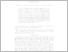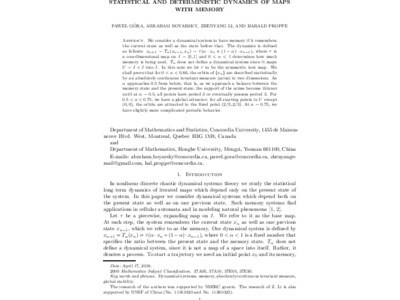Title:

# Statistical and Deterministic Dynamics of Maps with Memory

Gora, Pawel, Boyarsky, Abraham, Li, Zhenyang and Proppe, Harald (2017) Statistical and Deterministic Dynamics of Maps with Memory. Discrete and Continuous Dynamical System - A, 37 (8). pp. 4347-4378. ISSN ISSN: 1078-0947Preview Text (final draft) (application/pdf) Maps_with_memory12.pdf - Accepted Version Available under License Spectrum Terms of Access. 1MB

Official URL: http://dx.doi.org/10.3934/dcds.2017186

## Abstract

We consider a dynamical system to have memory if it remembers the current state as well as the state before that. The dynamics is defined as follows: $x_{n+1}=T_{\alpha }(x_{n-1},x_{n})=\tau (\alpha \cdot x_{n}+(1-\alpha )\cdot x_{n-1}),$ where $\tau$ is a one-dimensional map on $I=[0,1]$ and $0<\alpha <1$ determines how much memory is being used. $T_{\alpha}$ does not define a dynamical system since it maps $U=I\times I$ into $I$. In this note we let $\tau$ to be the symmetric tent map. We shall prove that
for $0<\alpha <0.46,$ the orbits of $\{x_{n}\}$ are described statistically by an absolutely continuous invariant measure (acim) in two dimensions. As $\alpha$ approaches $0.5$ from below, that is, as we approach a balance between the memory state and the present state, the support of the acims become thinner until at $\alpha=0.5$, all points have period 3 or eventually possess period 3. For $0.5<\alpha <0.75$, we have a global attractor: for all starting points in $U$ except $(0,0)$, the orbits are attracted to the fixed point $(2/3,2/3).$ At $\alpha=0.75,$ we have slightly more complicated periodic behavior.

Divisions: Concordia University > Faculty of Arts and Science > Mathematics and Statistics Article Yes Gora, Pawel and Boyarsky, Abraham and Li, Zhenyang and Proppe, Harald Discrete and Continuous Dynamical System - A August 2017 10.3934/dcds.2017186 985780 PAWEL GORA 03 Sep 2019 17:00 04 Sep 2019 00:00 Publication
All items in Spectrum are protected by copyright, with all rights reserved. The use of items is governed by Spectrum's terms of access.

Repository Staff Only: item control pageResearch related to the current document (at the CORE website)
Back to top